#1. 云栖社区>
2. 博客>
3. 正文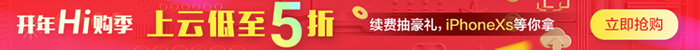# JSTL

jsp标准标签库（jstl）是一个JSP标签集合，它封装了jsp应用的通用核心功能。

JSTL支持通用的、格式化的任务。比如：迭代、条件判断、XML文档操作、国际化标签、SQL标签。除了这些它还提供了一个框架来使用集成JSTL的自定义标签。

### JSTL使用

<%@ taglib prefix="c" uri="http://java.sun.com/jsp/jstl/core" %>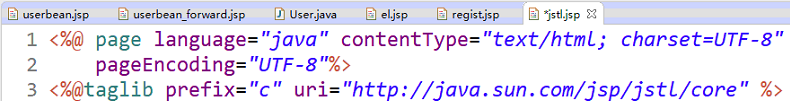if标签

<c:if>标签判断表达式的值，如果表达式的值为 true 则执行其主体内容。

<c:if test="<boolean>" var="<string>" scope="<string>"> ... </c:if>

<c:if>标签有如下属性：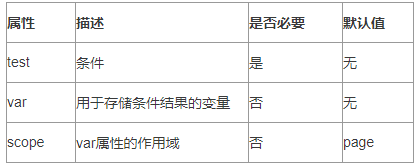``````<%@ page language="java" contentType="text/html; charset=UTF-8"
pageEncoding="UTF-8"%>
<%@ taglib uri="http://java.sun.com/jsp/jstl/core" prefix="c" %>
<html>
<title>c:if 标签实例</title>
<body>
<c:set var="salary" scope="session" value="\${2000*2}"/>
<c:if test="\${salary > 2000}">
<p>我的工资为: <c:out value="\${salary}"/><p>
</c:if>
</body>
</html>``````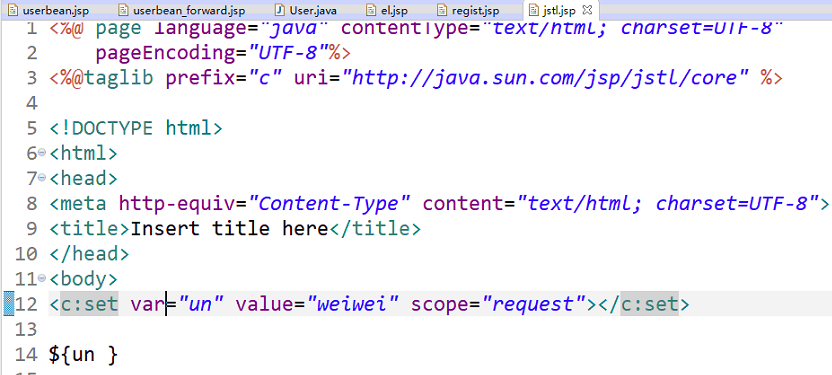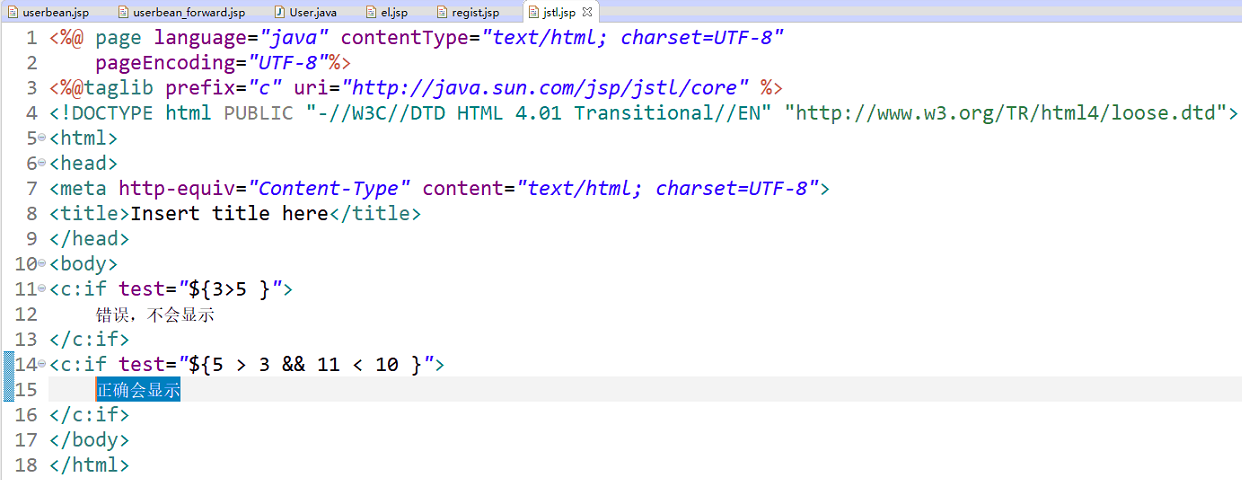### <c:choose>, <c:when>, <c:otherwise> 标签

<c:choose>标签与Java switch语句的功能一样，用于在众多选项中做出选择。

switch语句中有case，而<c:choose>标签中对应有<c:when>，switch语句中有default，而<c:choose>标签中有<c:otherwise>。

``````<c:choose>
<c:when test="<boolean>">
...
</c:when>
<c:when test="<boolean>">
...
</c:when>
...
...
<c:otherwise>
...
</c:otherwise>
</c:choose>``````

• <c:choose>标签没有属性。
• <c:when>标签只有一个属性，在下表中有给出。
• <c:otherwise>标签没有属性。

<c:when>标签的属性如下：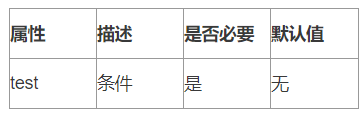``````<%@ page language="java" contentType="text/html; charset=UTF-8"
pageEncoding="UTF-8"%>
<%@ taglib uri="http://java.sun.com/jsp/jstl/core" prefix="c" %>
<html>
<title>c:choose 标签实例</title>
<body>
<c:set var="salary" scope="session" value="\${2000*2}"/>
<p>你的工资为 : <c:out value="\${salary}"/></p>
<c:choose>
<c:when test="\${salary <= 0}">
太惨了。
</c:when>
<c:when test="\${salary > 1000}">
不错的薪水，还能生活。
</c:when>
<c:otherwise>
什么都没有。
</c:otherwise>
</c:choose>
</body>
</html>``````

### <c:forEach>

<c:forEach>迭代一个集合中的对象。

forEach 语法格式

<c:forEach items="<object>" begin="<int>" end="<int>" step="<int>" var="<string>" varStatus="<string>"> 属性

<c:forEach>标签有如下属性：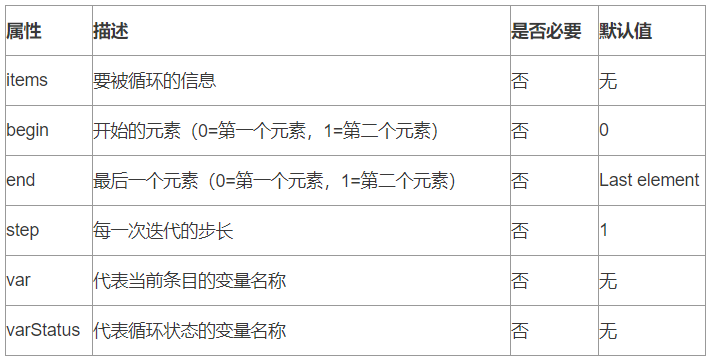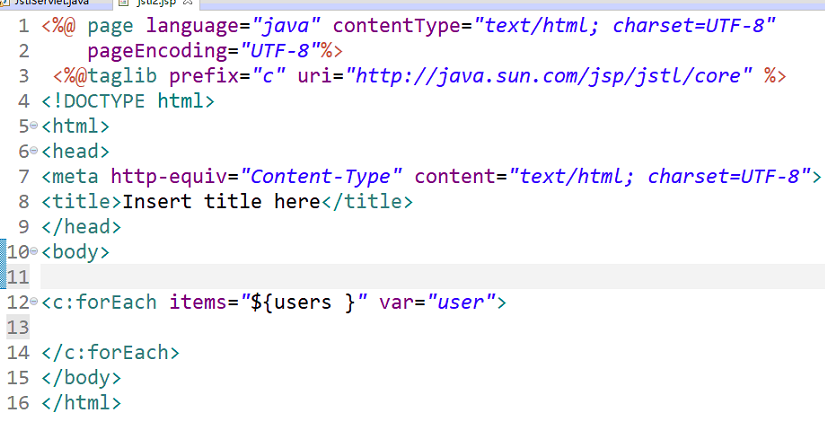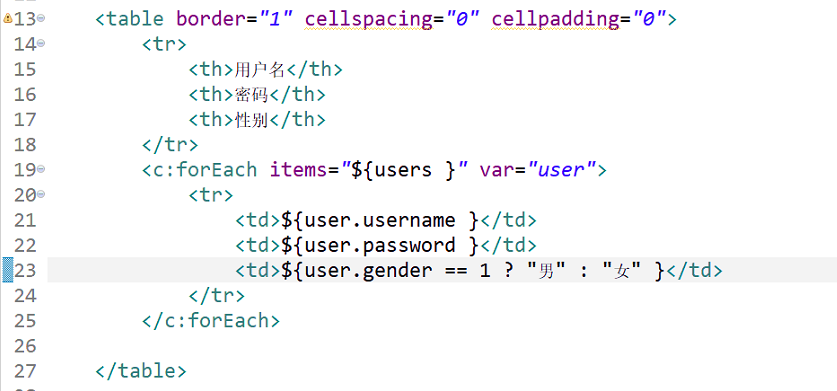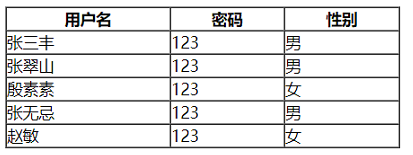【云栖快讯】一站式开发者服务，海量学习资源免费学  详情请点击

+ 关注